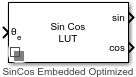# Sine-Cosine Lookup

Implement sine and cosine functions using lookup table approach

• Library:
• Motor Control Blockset / Controls / Math Transforms

•## Description

The Sine-Cosine Lookup block implements sine and cosine functions using the specified position or phase input signal.

The block uses the lookup table approach. This approach results in optimized code-execution when used with the model settings and configuration adopted by the examples shipped in Motor Control Blockset™. You can specify the number of lookup table points in the Number of data points for lookup table parameter.

This figure shows the input position and the generated sine and cosine output signals:## Ports

### Input

expand all

Position or phase value of the reference voltage signal specified as scalar in either per-unit, radians, or degrees.

Data Types: `single` | `double` | `fixed point`

### Output

expand all

Sine waveform output with a frequency that is identical to the position or phase signal (`θe`) frequency.

Data Types: `single` | `double` | `fixed point`

Cosine waveform output with a frequency that is identical to the position or phase signal (`θe`) frequency.

Data Types: `single` | `double` | `fixed point`

## Parameters

expand all

Unit of the input reference voltage position.

Size of the lookup table array.

## Version History

Introduced in R2020a